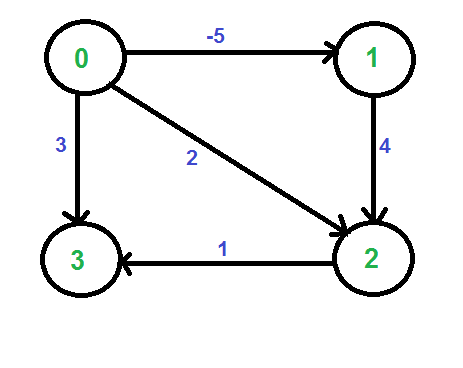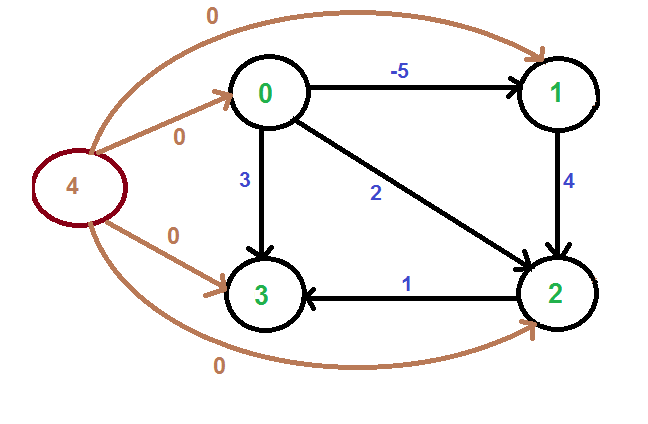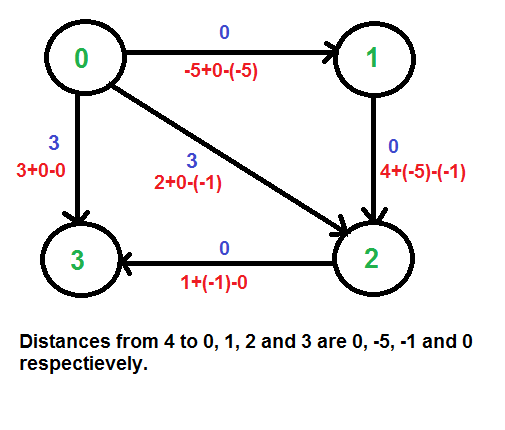Johnson’s algorithm for All-pairs shortest paths | Implementation

• Difficulty Level : Medium
• Last Updated : 12 Oct, 2018

Given a weighted Directed Graph where the weights may be negative, find the shortest path between every pair of vertices in the Graph using Johnson’s Algorithm.

The detailed explanation of Johnson’s algorithm has already been discussed in the previous post.

This post focusses on the implementation of Johnson’s Algorithm.

Recommended: Please try your approach on {IDE} first, before moving on to the solution.

Algorithm:

1. Let the given graph be G. Add a new vertex s to the graph, add edges from new vertex to all vertices of G. Let the modified graph be G’.
2. Run Bellman-Ford algorithm on G’ with s as source. Let the distances calculated by Bellman-Ford be h, h, .. h[V-1]. If we find a negative weight cycle, then return. Note that the negative weight cycle cannot be created by new vertex s as there is no edge to s. All edges are from s.
3. Reweight the edges of original graph. For each edge (u, v), assign the new weight as “original weight + h[u] – h[v]”.
4. Remove the added vertex s and run Dijkstra’s algorithm for every vertex.

Example:
Let us consider the following graph.We add a source s and add edges from s to all vertices of the original graph. In the following diagram s is 4.We calculate the shortest distances from 4 to all other vertices using Bellman-Ford algorithm. The shortest distances from 4 to 0, 1, 2 and 3 are 0, -5, -1 and 0 respectively, i.e., h[] = {0, -5, -1, 0}. Once we get these distances, we remove the source vertex 4 and reweight the edges using following formula. w(u, v) = w(u, v) + h[u] – h[v].Since all weights are positive now, we can run Dijkstra’s shortest path algorithm for every vertex as source.

Below is the implementation of the above approach

 # Implementation of Johnson's algorithm in Python3    # Import function to initialize the dictionary from collections import defaultdict MAX_INT = float('Inf')    # Returns the vertex with minimum  # distance from the source def minDistance(dist, visited):        (minimum, minVertex) = (MAX_INT, 0)     for vertex in range(len(dist)):         if minimum > dist[vertex] and visited[vertex] == False:             (minimum, minVertex) = (dist[vertex], vertex)        return minVertex       # Dijkstra Algorithm for Modified  # Graph (removing negative weights) def Dijkstra(graph, modifiedGraph, src):        # Number of vertices in the graph     num_vertices = len(graph)        # Dictionary to check if given vertex is      # already included in the shortest path tree     sptSet = defaultdict(lambda : False)        # Shortest distance of all vertices from the source     dist = [MAX_INT] * num_vertices        dist[src] = 0        for count in range(num_vertices):            # The current vertex which is at min Distance          # from the source and not yet included in the          # shortest path tree         curVertex = minDistance(dist, sptSet)         sptSet[curVertex] = True            for vertex in range(num_vertices):             if ((sptSet[vertex] == False) and                 (dist[vertex] > (dist[curVertex] +                  modifiedGraph[curVertex][vertex])) and                 (graph[curVertex][vertex] != 0)):                                    dist[vertex] = (dist[curVertex] +                                 modifiedGraph[curVertex][vertex]);        # Print the Shortest distance from the source     for vertex in range(num_vertices):         print ('Vertex ' + str(vertex) + ': ' + str(dist[vertex]))    # Function to calculate shortest distances from source # to all other vertices using Bellman-Ford algorithm def BellmanFord(edges, graph, num_vertices):        # Add a source s and calculate its min     # distance from every other node     dist = [MAX_INT] * (num_vertices + 1)     dist[num_vertices] = 0        for i in range(num_vertices):         edges.append([num_vertices, i, 0])        for i in range(num_vertices):         for (src, des, weight) in edges:             if((dist[src] != MAX_INT) and                      (dist[src] + weight < dist[des])):                 dist[des] = dist[src] + weight        # Don't send the value for the source added     return dist[0:num_vertices]    # Function to implement Johnson Algorithm def JohnsonAlgorithm(graph):        edges = []        # Create a list of edges for Bellman-Ford Algorithm     for i in range(len(graph)):         for j in range(len(graph[i])):                if graph[i][j] != 0:                 edges.append([i, j, graph[i][j]])        # Weights used to modify the original weights     modifyWeights = BellmanFord(edges, graph, len(graph))        modifiedGraph = [[0 for x in range(len(graph))] for y in                     range(len(graph))]        # Modify the weights to get rid of negative weights     for i in range(len(graph)):         for j in range(len(graph[i])):                if graph[i][j] != 0:                 modifiedGraph[i][j] = (graph[i][j] +                          modifyWeights[i] - modifyWeights[j]);        print ('Modified Graph: ' + str(modifiedGraph))        # Run Dijkstra for every vertex as source one by one     for src in range(len(graph)):         print ('\nShortest Distance with vertex ' +                         str(src) + ' as the source:\n')         Dijkstra(graph, modifiedGraph, src)    # Driver Code graph = [[0, -5, 2, 3],           [0, 0, 4, 0],           [0, 0, 0, 1],           [0, 0, 0, 0]]    JohnsonAlgorithm(graph)

Output:

Modified Graph: [[0, 0, 3, 3], [0, 0, 0, 0], [0, 0, 0, 0], [0, 0, 0, 0]]

Shortest Distance with vertex 0 as the source:

Vertex 0: 0
Vertex 1: 0
Vertex 2: 0
Vertex 3: 0

Shortest Distance with vertex 1 as the source:

Vertex 0: inf
Vertex 1: 0
Vertex 2: 0
Vertex 3: 0

Shortest Distance with vertex 2 as the source:

Vertex 0: inf
Vertex 1: inf
Vertex 2: 0
Vertex 3: 0

Shortest Distance with vertex 3 as the source:

Vertex 0: inf
Vertex 1: inf
Vertex 2: inf
Vertex 3: 0

Time Complexity: The time complexity of the above algorithm isas Dijkstra’s Algorithm takesfor adjacency matrix. Note that the above algorithm can be made more efficient by using adjacency list instead of the adjacency matrix to represent the Graph.

My Personal Notes arrow_drop_up
Recommended Articles
Page :